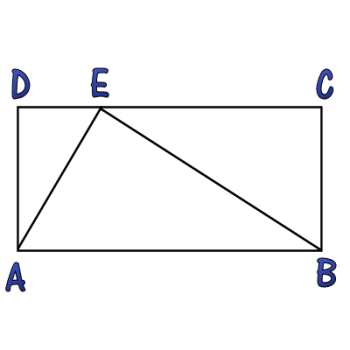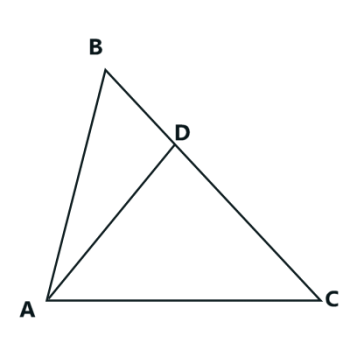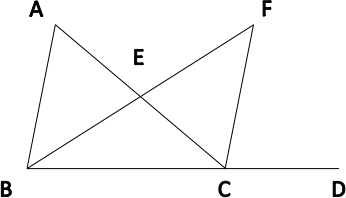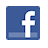Games
Problems
Go Pro!

# Triangle and Rectangle

Pro Problems > Math > Geometry > Triangles

## Triangle and RectangleIn the diagram displayed, the triangle splits the rectangle into three similar triangles. Find the value of AD2 divided by the product of DE and EC.

Presentation mode
Problem by Mr. H

## Solution

In order to make it feasible for teachers to use these problems in their classwork, no solutions are publicly visible, so students cannot simply look up the answers. If you would like to view the solutions to these problems, you must have a Virtual Classroom subscription.Assign this problem

## Similar Problems

### Forty-five and Thirty

Two of the angles of a triangle are 45 degrees and 30 degrees.  The altitude from the third angle has length 15 units. What is the triangle's area?

### Angle Riddle

The angles of a triangle meet the following criteria:

• All the angle measures are prime numbers.
• All the angle measures are distinct.
• Exactly two of my angle measures are palindromic.
• The difference between my two largest angle measures is a two digit number x.
• One of the digits of x is twice the other digit.

### Split Triangle

Segment AD is drawn in triangle ABC, with D on side BC. Given the following information, determine the measure of angle ADB.measure of angle BAD = x
measure of angle CAD = 2x
measure of angle ADC = 2y
measure of angle ABD = y
measure of angle ACD = x + y

### Angle Bisector

In the diagram shown,m ∠DCF = 80
m ∠ACF = 30
m ∠FBD = 50.

If segment BF bisects  ∠ABD, find m ∠BAC.

### Triangle in a Square

A triangle is placed on the interior of a square so that two of the triangle's vertices lie on two adjacent vertices of the square, and the third vertex lies on the opposite side,
1
3
of the way between the two endpoints.

If the perimeter of the triangle (in linear units) is equal to the area of the triangle (in square units), what is the length of the side of the square?

### Angle Measure

Line segment AB is intersected by segment DC, with D on AB, between A and B.

In triangle ADC, m∠ADC = 30º + x and  m∠DAC = 50º

In triangle ABC, m∠ACB = 115º + x

In triangle CDB, m∠CDB = 140º + x

Find m∠CBD.

### Angles in a Triangle

In a triangle, the measure of the largest angle is 12 less than the sum of the measures of the other two angles. The largest angle is also 52 more than the twice the middle angle decreased by three times the smallest angle. What is the largest angle measure?

The angles in a triangle have measures x2 - 5, 2x + 18, and x + 37. What is the measure of the largest angle in the triangle?

### Arithmetic Angles

The angles of a triangle are in arithmetic progression, and the difference between the largest and smallest angle is 42 degrees. What are the three angles?

### Three Triangles

The angles in a triangle have measures x, x + 10, and y.

The angles in a second triangle have measures x + y, 40, and 50.

The angles in a third triangle have measures x – y, 40, and z.

What is the value of z?

Two Triangles with Angle Measures, Triangle Variables, Three Two-Digit Angle Measures, Triangle in a Square# Featured Resources on This Site

Create Christmas ornaments and more
Print fun mazes in many different shapes

# Blogs on This SiteReviews and book lists - books we love!The site administrator fields questions from visitors.Like us on Facebook to get updates about new resources